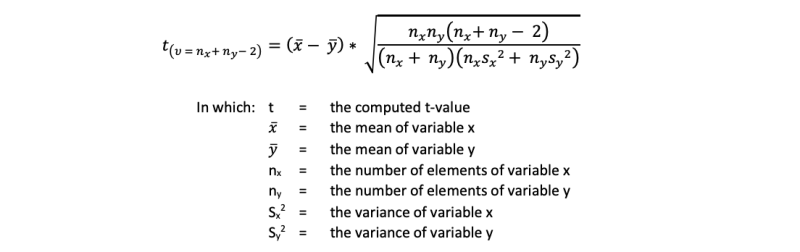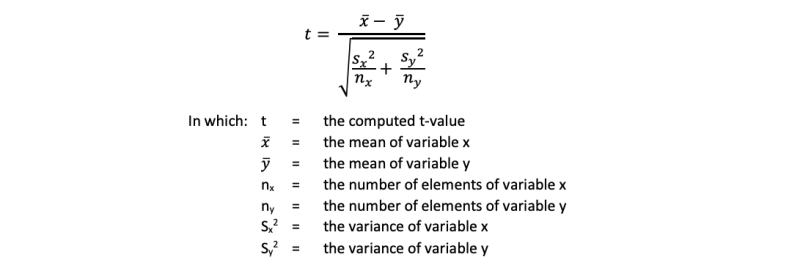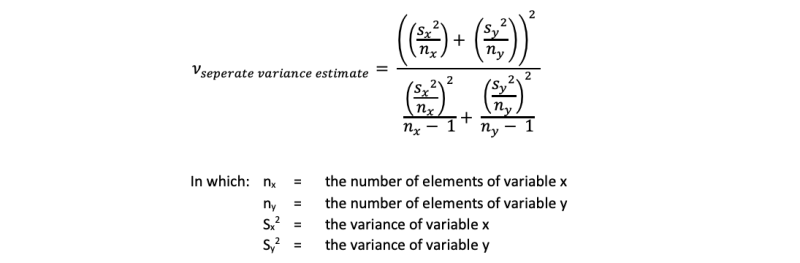t-test (groups)# If the road is not yet clear to you,we will show you the way how to get through.## t-test (groups)

### A t-test groups is a test for comparing the means of two groups.

There are different types of t-tests. The most common is a t-test between the means of two groups, and even of this type there are two subtypes. This is often called a t-test groups. This test will be discussed on this page.

Another well-known t-test is a test between the means of two characteristics. This is called a t-test pairs. This test is explained on a separate page.

Finally there is a t-test that tests if a computed mean (or value) differs from a standard. Very often authors refer to this test with one sample t-test. Most often the number 0 is used as the standard. This test is also explained on a separate page.

## t-test groups

This test is used for research questions like

Are males taller than females?Are there more sunny days a year in Sydney than in London?

Are there more sunny days a year in Sydney than in London?Do people in the European Union earn more money than in the United States?

Do people in the European Union earn more money than in the United States?

To apply the test you need to have a variable measured at a nominal level with two values - this is the independent variable – and a variable for which it is allowed to compute a mean – this is the dependent variable. A mean can be computed for variables measured at an interval or ratio level. In our first research question the independent variable is gender (males versus females) and the dependent variable is length. In the second example the independent variable is place (Sydney versus London) and the dependent variable is sunny days (for a great many years). In the third example the independent variable is place (European Union versus United States) and the dependent variable is income.

After detecting the right variables, for each group the mean and the standard deviation can be computed and the number of elements can be counted. If both groups do not differ much on the number of elements and the standard deviation, then this formula can be used to compare the means:However, if the standard deviations or the numbers of cases in both groups are very different, then this formula has to be applied:Though this formula is simpler than the first, the degrees of freedom are more difficult to establish. For the first formula the degrees of freedom are equal to the number of elements of both variables minus 2 (n(x) + n(y) – 2), but for the second one you need to fill in this formula:Anyhow, when you have computed the t-value and the degrees of freedom, you are able to compare this calculated t-value with a critical t-value. The critical values can be found in any good textbook about statistics or in Excel. If the computed t-value exceeds the critical t-value, it is said that the test is statistically significant. If this is abracadabra to you, please read our paper about the statistical test procedure where this is explained in detail.

The t-test groups can be applied to minimal 30 elements in each group - so 60 in total - and the dependent variable should allow you to compute a mean rather well. If your data does not meet these criteria, a Mann-Whitney test should be used.

An ANOVA can be applied too. The ANOVA is obliged when there are 3 or more groups; the t-test is normally used for two groups. But if you calculate an ANOVA with two groups you get the same results as in the first formula above.

Use our SPSS tutorials to learn how to run this test in SPSS.

## Mission

My goal is to teach you how to conduct good research.

Because:

Good research provides you with better information.
With better information, you can make better decisions.
With better decisions, you can create a healthier, wealthier and freer world,
for people, fauna and flora, for current and future generations.

That is why I think it is important that  you know how to do your research well.